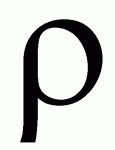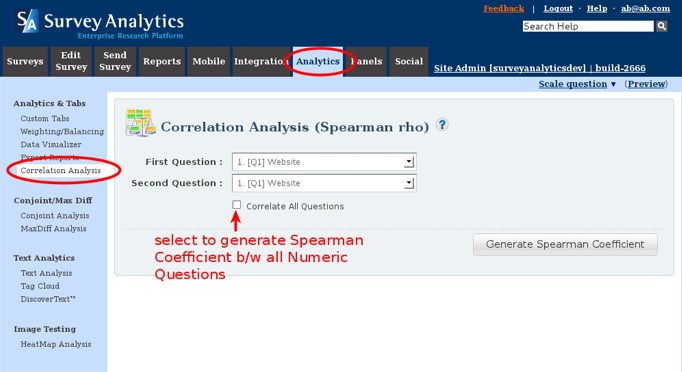### Survey Analytics Adds Spearman’s Rho Correlation Coefficient

Survey Analytics’ Enterprise Research Platform has introduced a powerful way to measure of correlation between two variables, the so-called Spearman’s rho correlation coefficient.

[caption id="attachment_2617" align="aligncenter" width="115"]The Greek Letter rho[/caption]

A correlation coefficient is a number between -1 and +1 which describes the extent to which two variables are related. Values near 1 indicate a strong positive association; values near -1 indicate a strong negative (or inverse) association; and values near 0 indicate a lack of correlation altogether.

Spearman’s rho is a non-parametric statistic, meaning it does not assume any particular distribution of the variables being described. Other common correlation coefficient statistics, notably Pearson’s r, assume the variables are distributed normally.

Survey Analytics plans to add the Pearson’s r correlation coefficient in another upcoming release.

Click here for a tutorial on how to calculate Spearman’s rho using Survey Analytics’ Enteprise Research Platform.